# SPECTRAL PEAK LOCATION ALGORITHM

In the practical world of discrete spectrum analysis, we often want to estimate the frequency of a sinusoid (or the center frequency of a very narrowband signal of interest). Upon applying the radix-2 fast Fourier transform (FFT), our narrowband signals of interest rarely reside exactly on an FFT bin center whose frequency is exactly known. As such, due to the FFT's leakage properties, the discrete spectrum of a sinusoid having N time-domain samples may look like the magnitude samples shown in Figure 13-37(a). There we see the sinusoid's spectral peak residing between the FFT's m = 5 and m = 6 bin centers. (Variable m is an N-point FFT's frequency-domain index. The FFT bin spacing is fs/N where, as always, fs is the sample rate.) Close examination of Figure 13-37(a) allows us to say the sinusoid lies in the range of m = 5 and m = 5.5, because we see that the maximum spectral sample is closer to the m = 5 bin center than the m = 6 bin center. The real-valued sinusoidal time signal has, in this example, a frequency of 5.25fs/N Hz. In this situation, our frequency estimation resolution is half the FFT bin spacing. We often need better frequency estimation resolution, and there are indeed several ways to improve that resolution.

Figure 13-37. Spectral magnitudes: (a) N-point FFT; (b) 4N-point FFT.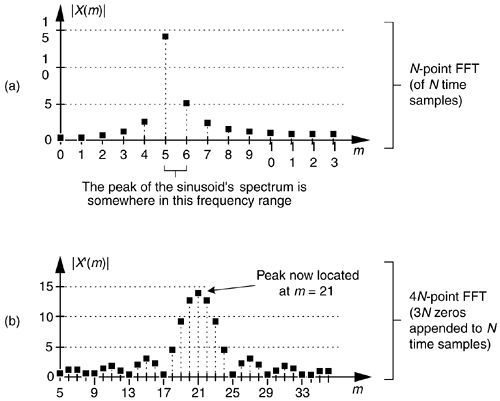We could collect, say, 4N time-domain signal samples and perform a 4N-point FFT yielding a reduced bin spacing of fs/4N. Or we could pad (append to the end of the original time samples) the original N time samples with 3N zero-valued samples and perform a 4N-point FFT on the lengthened time sequence. That would also provide an improved frequency resolution of fs/4N, as shown in Figure 13-37(b). With the spectral peak located at bin mpeak = 21, we estimate the signal's center frequency, in Hz, using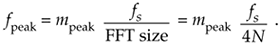Both schemes, collect more data and zero-padding, are computationally expensive. Many other techniques for enhanced-precision frequency measurement have been described in the scientific literature—from the close-to-home field of geophysics to the lofty studies of astrophysics—but most of those schemes seek precision without regard to computational simplicity. Here we describe a computationally simple frequency estimation scheme.

Assume we have FFT spectral samples X(m), of a real-valued narrowband time signal, whose magnitudes are shown in Figure 13-38. The vertical magnitude axis is linear, not logarithmic.

Figure 13-38. FFT spectral magnitudes of a narrowband signal.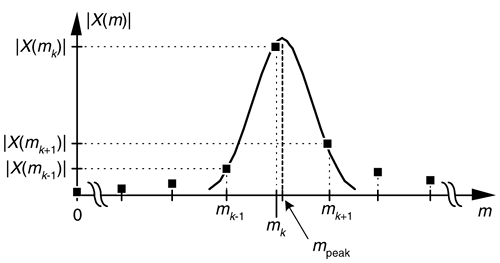The signal's index-based center frequency, mpeak, can be estimated using

Equation 13-73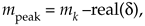where real(d) means the real part of the d correction factor defined as

Equation 13-74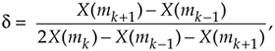where mk is the integer index of the largest magnitude sample |X(mk)|. Values X(mk–1) and X(mk+1) are the complex spectral samples on either side of the peak sample as shown in Figure 13-38. Based on the complex spectral values, we compute the signal's index-based frequency mpeak (which may not be an integer), and apply that value to

Equation 13-75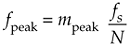to provide a frequency estimate in Hz. Equations (13-73) and (13-74) apply only when the majority of the signal's spectral energy lies within a single FFT bin width (fs/N).

This spectral peak location estimation algorithm is quite accurate for its simplicity. Its peak frequency estimation error is roughly 0.06, 0.04, and 0.03 bin widths for signal-to-noise ratios of 3, 6, and 9 dB respectively. Not bad at all! The nice features of the algorithm are that it does not require the original time samples to be windowed, as do some other spectral peak location algorithms, and it uses the raw FFT samples without the need for spectral magnitudes to be computed.

URL http://proquest.safaribooksonline.com/0131089897/ch13lev1sec15AmazonPrev don't be afraid of buying books Next